# Magnitude (mathematics) facts for kids

Kids Encyclopedia Facts

The magnitude of a mathematical object is its size: a property by which it can be larger or smaller than other objects of the same kind.

In mathematical language one would say: It is an ordering of the class of objects to which it belongs.

The Ancient Greeks distinguished between several types of magnitude, including:

They had proven that the first two could not be the same, or even isomorphic systems of magnitude. They did not consider negative magnitudes to be meaningful, and magnitude is still primarily used in contexts in which zero is either the lowest size, or less than all possible sizes.

## Real numbers

The magnitude of a real number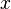$x$ is usually called the absolute value or modulus. It is written as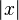$|x|$, and is defined by:

| x | = x, if x ≥ 0
| x | = -x, if x < 0

This gives the number's distance from zero on the real number line. For example, the modulus of -5 is 5.

## Vector

The magnitude of a vector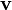$\mathbf{v}$ is called its norm, and is usually written as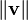$\|\mathbf{v}\|$. It measures the length of the vector. For a three-dimensional vector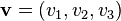$\mathbf{v} = (v_1, v_2, v_3)$, the norm can be calculated using the formula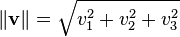$\|\mathbf{v}\| = \sqrt{v_1^2 + v_2^2 + v_3^2}$.

## Practical math

A magnitude is never negative. When comparing magnitudes, it is often helpful to use a logarithmic scale. Real-world examples include the loudness of a sound (decibel), the brightness of a star, or the Richter scale of earthquake intensity.

Because magnitudes are often not linear, they usually cannot be added or subtract in a meaningful way.

## Related pagesMagnitude (mathematics) Facts for Kids. Kiddle Encyclopedia.